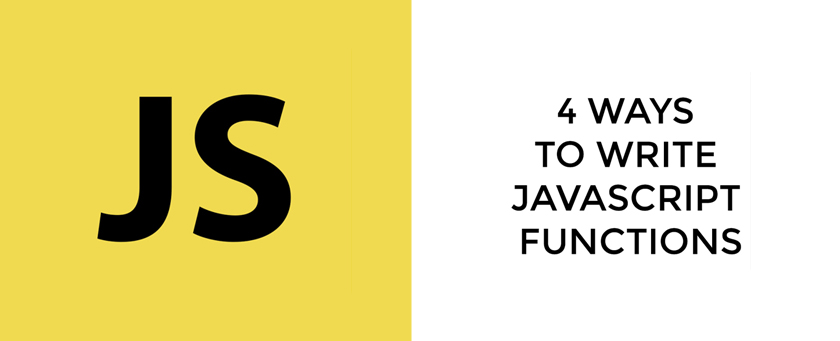# Four Different Ways to Write Functions in JavascriptFunctions are the basic building blocks of the Javascript. A function in Javascript is a code block that performs a specific task or set of tasks.

There are basically 4 (four) different ways we can define functions in Javascript.

• Function Declaration
• Function Expression
• Arrow Function Expression
• Concise Arrow Function Expression
// Function Declaration
function square(x) {
return x * x;
}

// Function Expression
const square = function(x) {
return x * x;
}

// Arrow Function Expression
const square = x => {
return x * x;
}

// Concise Arrow Function Expression
const square = x => x * x;


If you prefer you can take a look at the video tutorial,